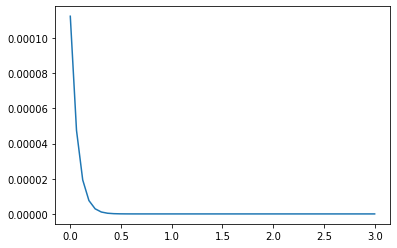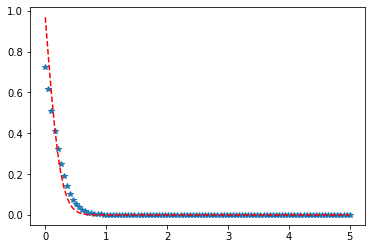# Python – Johnson SU Distribution in Statistics

scipy.stats.johnsonsu() is a Johnson SU continuous random variable that is defined with a standard format and some shape parameters to complete its specification.

Parameters :

q : lower and upper tail probability
x : quantiles
loc : [optional]location parameter. Default = 0
scale : [optional]scale parameter. Default = 1
size : [tuple of ints, optional] shape or random variates.
moments : [optional] composed of letters [‘mvsk’]; ‘m’ = mean, ‘v’ = variance, ‘s’ = Fisher’s skew and ‘k’ = Fisher’s kurtosis. (default = ‘mv’).

Results : Johnson SU continuous random variable

Code #1 : Creating Johnson SU continuous random variable

 `# importing library ` ` `  `from` `scipy.stats ``import` `johnsonsu   ` `   `  `numargs ``=` `johnsonsu.numargs  ` `a, b ``=` `4.32``, ``3.18` `rv ``=` `johnsonsu(a, b)  ` `   `  `print` `(``"RV : \n"``, rv)   `

Output :

```RV :
scipy.stats._distn_infrastructure.rv_frozen object at 0x000002A9D4E91708
```

Code #2 : Johnson SU continuous variates and probability distribution

 `import` `numpy as np  ` `quantile ``=` `np.arange (``0.01``, ``1``, ``0.1``)  ` ` `  `# Random Variates  ` `R ``=` `johnsonsu.rvs(a, b, scale ``=` `2``, size ``=` `10``)  ` `print` `(``"Random Variates : \n"``, R)  ` ` `  `# PDF  ` `R ``=` `johnsonsu.pdf(a, b, quantile, loc ``=` `0``, scale ``=` `1``)  ` `print` `(``"\nProbability Distribution : \n"``, R)   `

Output :

```Random Variates :
[-6.33841843 -5.35469028 -5.36145351 -4.4504208  -1.91574847 -5.01633416
-5.37699657 -4.15794134 -4.90450547 -2.93846617]

Probability Distribution :
[5.34745702e-06 2.86846536e-05 2.54767528e-05 1.66921608e-05
9.34800722e-06 4.69729578e-06 2.16525150e-06 9.26607636e-07
3.70800055e-07 1.39402846e-07]
```

Code #3 : Graphical Representation.

 `import` `numpy as np  ` `import` `matplotlib.pyplot as plt  ` `    `  `distribution ``=` `np.linspace(``0``, np.minimum(rv.dist.b, ``3``))  ` `print``(``"Distribution : \n"``, distribution)  ` `    `  `plot ``=` `plt.plot(distribution, rv.pdf(distribution))  `

Output :

```Distribution :
[0.         0.06122449 0.12244898 0.18367347 0.24489796 0.30612245
0.36734694 0.42857143 0.48979592 0.55102041 0.6122449  0.67346939
0.73469388 0.79591837 0.85714286 0.91836735 0.97959184 1.04081633
1.10204082 1.16326531 1.2244898  1.28571429 1.34693878 1.40816327
1.46938776 1.53061224 1.59183673 1.65306122 1.71428571 1.7755102
1.83673469 1.89795918 1.95918367 2.02040816 2.08163265 2.14285714
2.20408163 2.26530612 2.32653061 2.3877551  2.44897959 2.51020408
2.57142857 2.63265306 2.69387755 2.75510204 2.81632653 2.87755102
2.93877551 3.        ]
```Code #4 : Varying Positional Arguments

 `import` `matplotlib.pyplot as plt  ` `import` `numpy as np  ` `    `  `x ``=` `np.linspace(``0``, ``5``, ``100``)  ` `    `  `# Varying positional arguments  ` `y1 ``=` `johnsonsu .pdf(x, ``1``, ``3``)  ` `y2 ``=` `johnsonsu .pdf(x, ``1``, ``4``)  ` `plt.plot(x, y1, ``"*"``, x, y2, ``"r--"``)  `

Output :My Personal Notes arrow_drop_upCheck out this Author's contributed articles.

If you like GeeksforGeeks and would like to contribute, you can also write an article using contribute.geeksforgeeks.org or mail your article to contribute@geeksforgeeks.org. See your article appearing on the GeeksforGeeks main page and help other Geeks.

Please Improve this article if you find anything incorrect by clicking on the "Improve Article" button below.

Article Tags :

Be the First to upvote.

Please write to us at contribute@geeksforgeeks.org to report any issue with the above content.